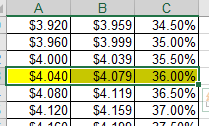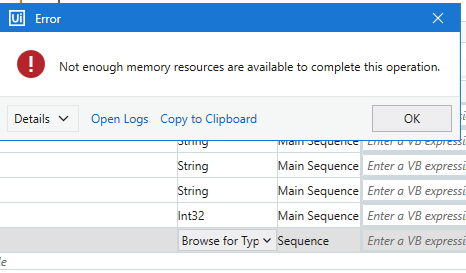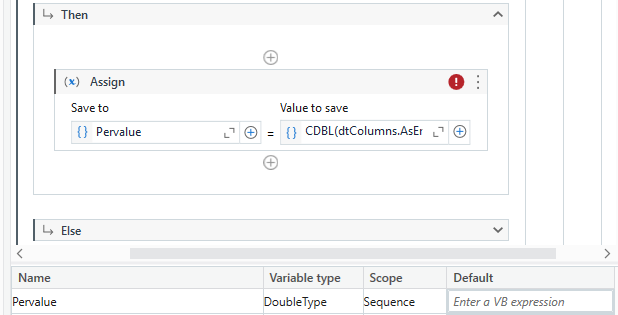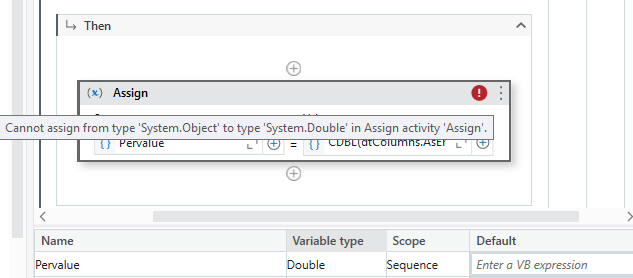# How to compare a value in the range of two columns in excel

Hi
I have some value and that value has to be found between range of data available in first two columns of excel. If the range is found, then the data available in next column has to be saved.

my value is 4.052, so this value is present in the range of below highlighted row and i need to get the value present in column C that is 36%. Some one please assist.1. Read the range into datatable dt
2. `Dt.AsEnumerable.Where(function(x) valuevar>Cint(x(0)) And Cint(x(1)) > valuevar).count>0` use this in if condition
3. On then side use assign to get the percentage (double type) `percentvar = CDBL(Dt.AsEnumerable.Where(function(x) valuevar>Cint(x(0)) And Cint(x(1)) > valuevar)(0)(3))`

Cheers

Hi @Anil_G , Thanks for the reply. When I tried to change the variable type, I’m getting below error. This never happened.Hi, i got variable type as “DoubleType” but there is an error as shown below.Type is `system.double`

Cheers

hi @Anil_G, after changing to system.double, I’m getting same error.`percentvar = CDBL(Dt.AsEnumerable.Where(function(x) valuevar>Cint(x(0)) And Cint(x(1)) > valuevar)(0)(3).ToString)`